# SBI Clerk Prelims Reasoning Ability Questions 2021 (Day-01)

Dear Aspirants, Our IBPS Guide team is providing new series of Reasoning Questions for SBI Clerk Prelims 2021 so the aspirants can practice it on a daily basis. These questions are framed by our skilled experts after understanding your needs thoroughly. Aspirants can practice these new series questions daily to familiarize with the exact exam pattern and make your preparation effective.

Start Quiz

Square based seating arrangement

Direction (1-5): Study the following information carefully and answer the below questions.

Eight persons-P, Q, R, S, T, U, V, and W are sitting in the square table facing outside. Four persons are sitting in the middle of the table while four persons are sitting at the corner of the table.

T sits at the corner of the table. U sits opposite to T. P and U are sitting adjacent to each other. Q sits at the corner of the table but not adjacent to P. W sits adjacent to neither T nor U. Two persons are sitting between S and W. S sits adjacent to either T or P. R sits second to the left of S. V is not an immediate neighbour of W.

1) Who among the following person sits third to the right of T?

A.V

B.The one who sits immediate right of Q

C.The one who sits immediate left of U

D.The one who sits third to the left of W

E.All of the above

2) Who among the following person sits exactly between R and S when counted from the right of R?

A.The person who sits second to the right of W

B.V

C.The person who sits to the immediate right of Q

D.P

E.None of the above

3) Which of the following statement is/are true?

A.Q and W are sitting opposite to each other

B.Only one person sits between U and Q when counted from the left of U.

C.T sits second to the left of Q

D.R and W are sitting adjacent to each other.

E.All are true

4) Which of the following combination of persons are immediate neighbours?

A.TQ

B.WU

C.UV

D.PR

E.PS

5) Four of the following five are alike in a certain way thus forms a group. Find the odd one which does not belong to the group?

A.Q

B.T

C.W

D.U

E.P

Inequality

Direction (6-10): In each of the following questions, the relationship between different elements is shown in the statements followed by two conclusions. Find the conclusion which logically follows.

6) Statements:

M < F < H = O;   L < K = T ≥ H; O > V = P

Conclusions:

I). L < P

II).F > T

A.If only conclusion I follows.

B.If only conclusion II follows.

C.If either conclusion I or II follows.

D.If neither conclusion I nor II follows.

E.If both conclusions I and II follow.

7) Statements:

M < A ≤ Q > Z > P; Y < T = F ≤ M

Conclusions:

I). Q > T

II). F < P

A.If only conclusion I follows.

B.If only conclusion II follows.

C.If either conclusion I or II follows.

D.If neither conclusion I nor II follows.

E.If both conclusions I and II follow.

8) Statements:

C < R < X = J < Z; Q > K > Z; E ≤ M > P < Q

Conclusions:

I). M ≤ Z

II). C < Q

A.If only conclusion I follows.

B.If only conclusion II follows.

C.If either conclusion I or II follows.

D.If neither conclusion I nor II follows.

E.If both conclusions I and II follow.

9) Statements:

B < C < T ≤ R = Q; F > P > G = I > Q

Conclusions:

I). R < G

II). T < P

A.If only conclusion I follows.

B.If only conclusion II follows.

C.If either conclusion I or II follows.

D.If neither conclusion I nor II follows.

E.If both conclusions I and II follow.

10) Statements:

Q < X ≤ H ≤ K ≤ M; W ≥ L = E ≥ M

Conclusions:

I). X < L

II). X = L

A.If only conclusion I follows.

B.If only conclusion II follows.

C.If either conclusion I or II follows.

D.If neither conclusion I nor II follows.

E.If both conclusions I and II follow.

Directions (1-5) :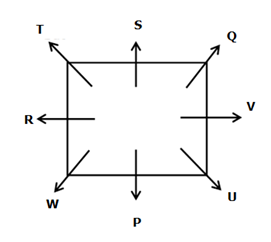P and U are sitting adjacent to each other.

U sits opposite to T.

T sits at the corner of the table.

From the above condition, there are two possibilities.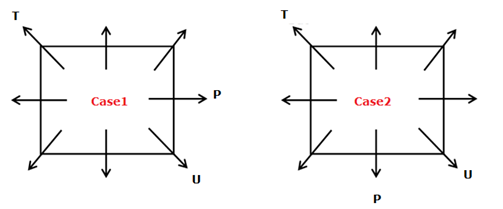Q sits at the corner of the table but not adjacent to P.

W sits adjacent to neither T nor U.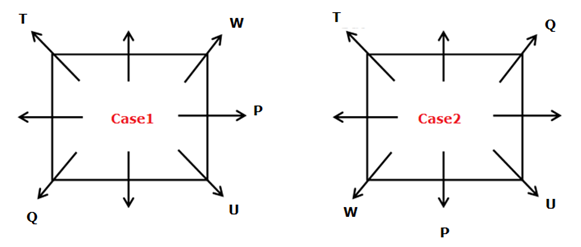Two persons are sitting between S and W.

S sits adjacent to either T or P.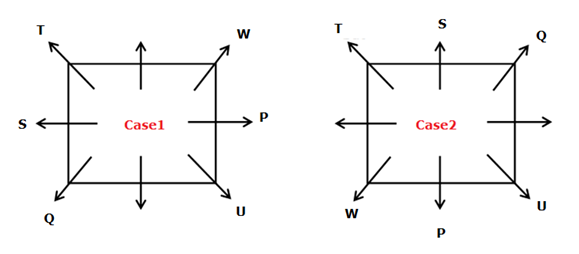R sits second to the left of S.

V is not an immediate neighbour of W.

From the above condition, case1 gets eliminated. Case-2 shows the final arrangement.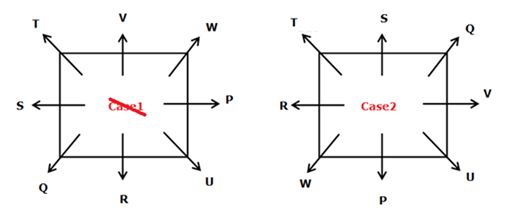Directions (6-10) :

I). L < P (L < K = T ≥ H = O > V= P) -> False

II). F > T (F < H ≤ T) -> False

Neither conclusion I nor II follows.

I). Q > T (Y < T = F ≤ M < A ≤ Q) -> True

II). F < P (F ≤ M < A ≤ Q > Z >P) -> False

Only conclusion I follow.

I). M ≤ Z (M > P< Q > K > Z) -> False

II). C < Q (C < R < X = J < Z < K < Q) -> True

Only conclusion II follows

I). R < G (G = I > Q = R) -> True

II). T < P (P > G = I > Q = R ≥ T) -> True

Both conclusions I and II follow.